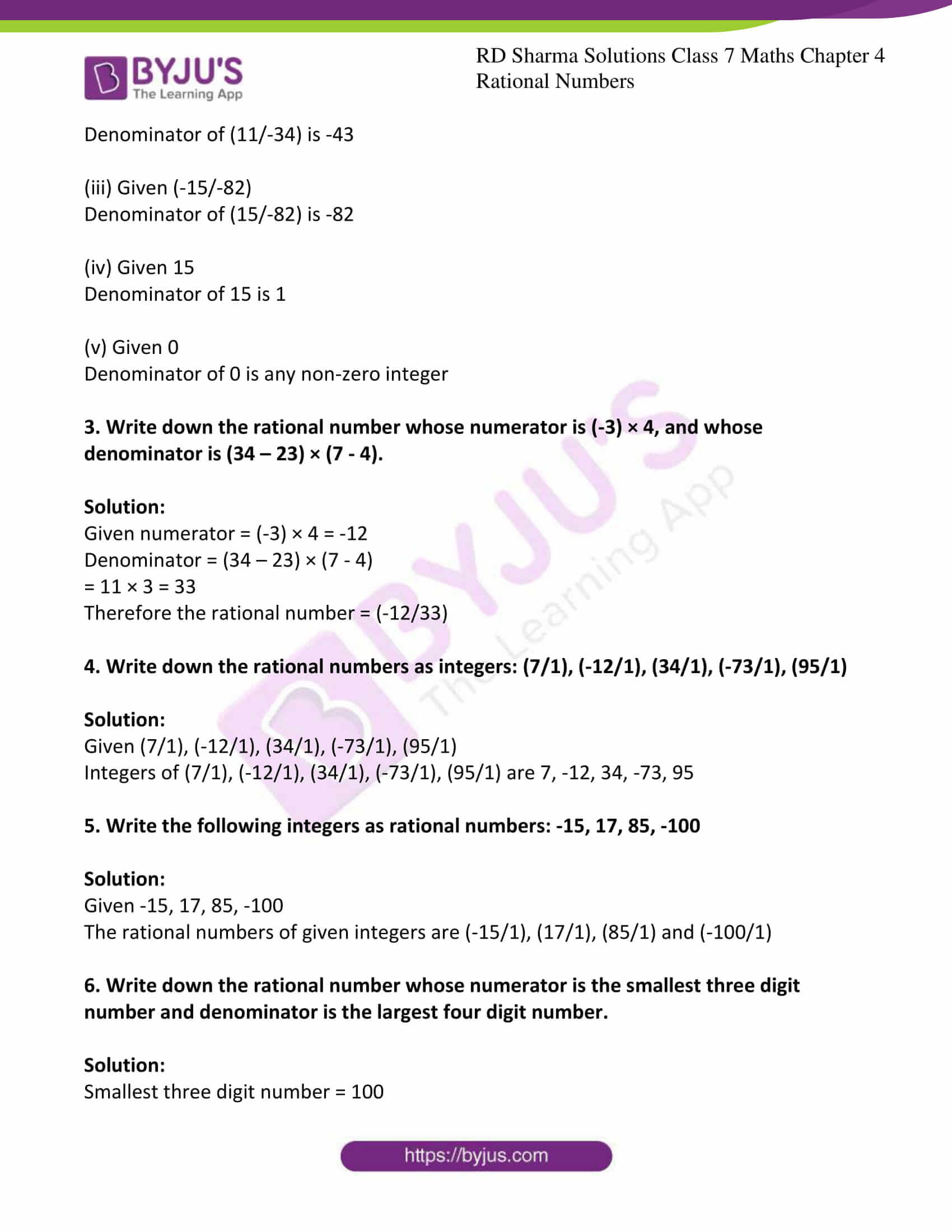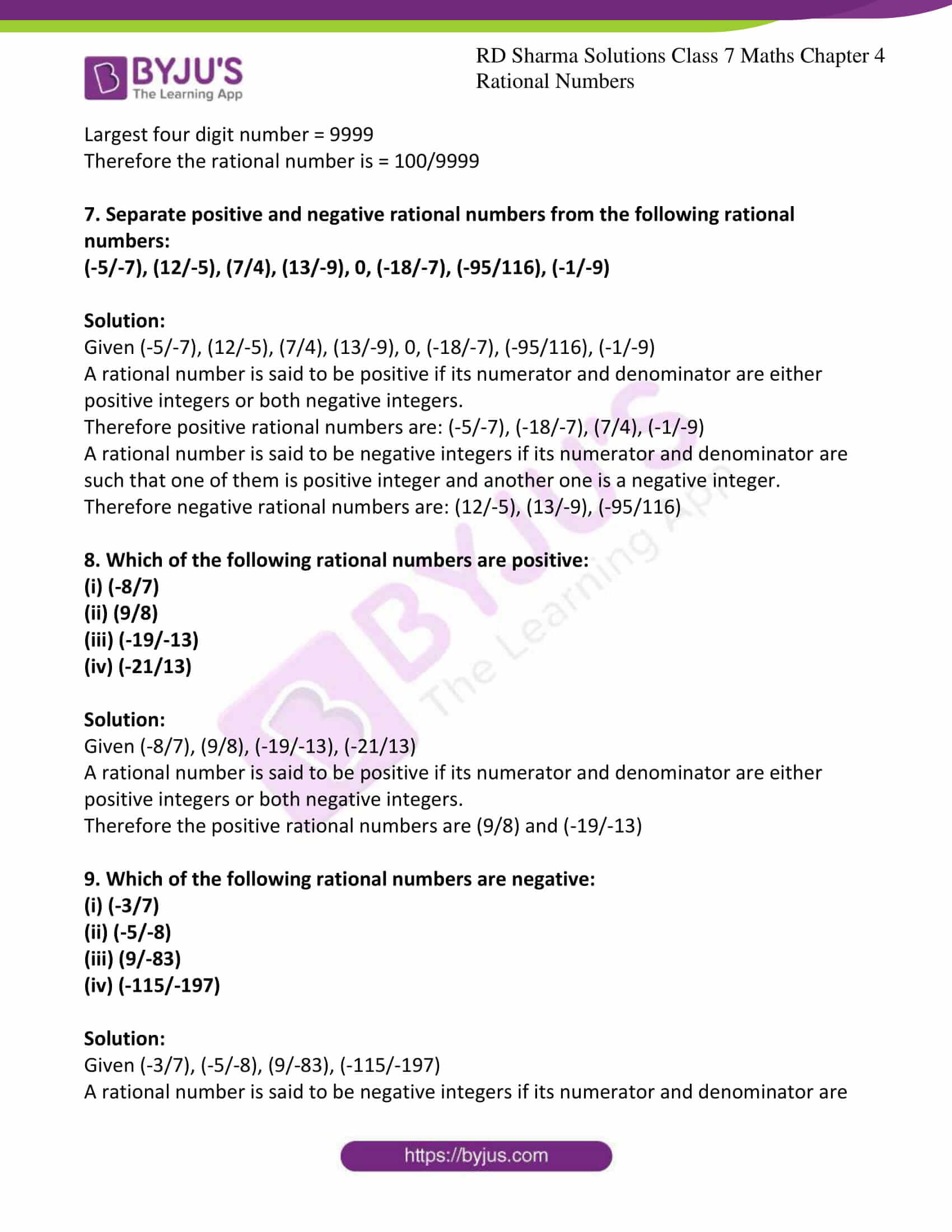# RD Sharma Solutions For Class 7 Maths Exercise 4.1 Chapter 4 Rational Numbers

RD Sharma Solutions for Class 7 Exercise 4.1 of Chapter 4 Rational Numbers is the best study material for those students who are finding difficulties in solving math problems. Students can download the pdf of RD Sharma Solutions for Class 7 Maths Chapter 4 Rational Numbers from the available links. The math problems present in this textbook is formulated by subject experts at BYJU’S to reduce the burden of students.

A number which can be expressed in the form p/q, where p and q are integers and q not equal to zero are called rational numbers. Some of the topics discussed in this exercise are listed below.

• Definition and meaning of rational numbers
• Properties of rational numbers
• The numerator and denominator of rational numbers
• Positive rational numbers
• Negative rational numbers

## Download the PDF of RD Sharma Solutions For Class 7 Maths Chapter 4 – Rational Numbers Exercise 4.1### Access answers to Maths RD Sharma Solutions For Class 7 Chapter 4 – Rational Numbers Exercise 4.1

1. Write down the numerator of each of the following rational numbers:

(i) (-7/5)

(ii) (14/-4)

(iii) (-17/-21)

(iv) (8/9)

(v) 5

Solution:

(i) Given (-7/5)

Numerator of (-7/5) is -7

(ii) Given (14/-4)

Numerator of (14/-4) is 1

(iii) Given (-17/-21)

Numerator of (-17/-21) is -17

(iv) Given (8/9)

Numerator of (8/9) is 8

(v) Given 5

Numerator of 5 is 5

2. Write down the denominator of each of the following rational numbers:

(i) (-4/5)

(ii) (11/-34)

(iii) (-15/-82)

(iv) 15

(v) 0

Solution:

(i) Given (-4/5)

Denominator of (-4/5) is 5

(ii) Given (11/-34)

Denominator of (11/-34) is -43

(iii) Given (-15/-82)

Denominator of (15/-82) is -82

(iv) Given 15

Denominator of 15 is 1

(v) Given 0

Denominator of 0 is any non-zero integer

3. Write down the rational number whose numerator is (-3) × 4, and whose denominator is (34 – 23) × (7 – 4).

Solution:

Given numerator = (-3) × 4 = -12

Denominator = (34 – 23) × (7 – 4)

= 11 × 3 = 33

Therefore the rational number = (-12/33)

4. Write down the rational numbers as integers: (7/1), (-12/1), (34/1), (-73/1), (95/1)

Solution:

Given (7/1), (-12/1), (34/1), (-73/1), (95/1)

Integers of (7/1), (-12/1), (34/1), (-73/1), (95/1) are 7, -12, 34, -73, 95

5. Write the following integers as rational numbers: -15, 17, 85, -100

Solution:

Given -15, 17, 85, -100

The rational numbers of given integers are (-15/1), (17/1), (85/1) and (-100/1)

6. Write down the rational number whose numerator is the smallest three digit number and denominator is the largest four digit number.

Solution:

Smallest three digit number = 100

Largest four digit number = 9999

Therefore the rational number is = 100/9999

7. Separate positive and negative rational numbers from the following rational numbers:

(-5/-7), (12/-5), (7/4), (13/-9), 0, (-18/-7), (-95/116), (-1/-9)

Solution:

Given (-5/-7), (12/-5), (7/4), (13/-9), 0, (-18/-7), (-95/116), (-1/-9)

A rational number is said to be positive if its numerator and denominator are either positive integers or both negative integers.

Therefore positive rational numbers are: (-5/-7), (-18/-7), (7/4), (-1/-9)

A rational number is said to be negative integers if its numerator and denominator are such that one of them is positive integer and another one is a negative integer.

Therefore negative rational numbers are: (12/-5), (13/-9), (-95/116)

8. Which of the following rational numbers are positive:

(i) (-8/7)

(ii) (9/8)

(iii) (-19/-13)

(iv) (-21/13)

Solution:

Given (-8/7), (9/8), (-19/-13), (-21/13)

A rational number is said to be positive if its numerator and denominator are either positive integers or both negative integers.

Therefore the positive rational numbers are (9/8) and (-19/-13)

9. Which of the following rational numbers are negative:

(i) (-3/7)

(ii) (-5/-8)

(iii) (9/-83)

(iv) (-115/-197)

Solution:

Given (-3/7), (-5/-8), (9/-83), (-115/-197)

A rational number is said to be negative integers if its numerator and denominator are such that one of them is positive integer and another one is a negative integer.

Therefore negative rational numbers are (-3/7) and (9/-83)